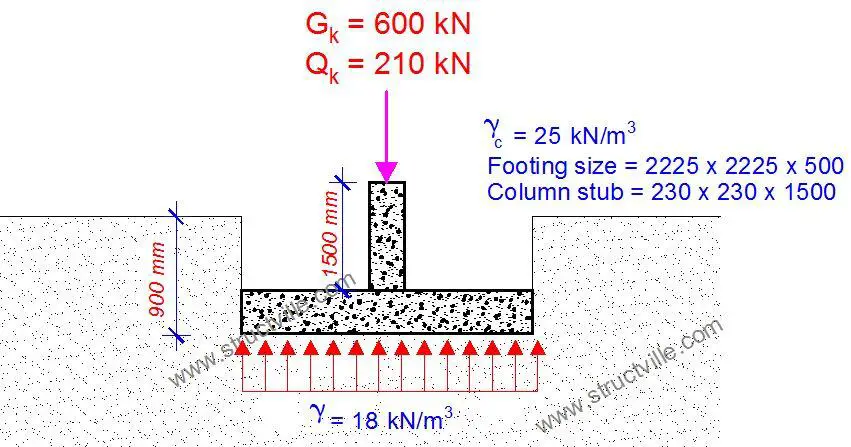# Question of the Day | 27-12-2020The 2225 mm x 2225 mm pad footing shown above is meant to support a 230 x 230 mm column from the superstructure of a building. The characteristic dead and imposed loads on the column are 600 kN and 210 kN respectively, excluding the weight of the column stub. Making use of your personal engineering judgment, kindly answer the following questions using the information provided;

(1) Will you accept the size of the pad footing provided if the verified allowable bearing capacity of the soil is 175 kN/m2?

(2) What is your calculated value of the total service load for determining the footing dimensions?

(3) What informed your decision? Did you make any assumptions?

1.Shubham Kanojiya

Self weight of column = (0.23*0.23*1.5*25)=1.98kN
Self weight of footing = (2.25*2.25*0.5*25)=63.28kN
Weigh of soil above footing = ((2.25*2.25)-(0.23*0.23))*(0.9-0.5)*18= 36kN
Minimum Area required = 911.26/175 = 5.21sq.m
Area provided = (2.25*2.25)= 5.06sq.m

•Karsten Iversen

In Den ark, we have two scenario’s to look at. 1. With G multiplied with 1,2 and no Q. The se ond scenario is G+1,5Q and we normallu use Concretes load at 24 kN/m3.
1st scenario 600 * 1,2 + 1,90 + 60,74 + 36 = 1006 kN which is a load under structure on 198,9 kN/m2
2nd scenario 600+210*1,5+1,90+60,74+36 = 1013,6 kN (a little bit higher) which gives the result under the plate 200,2 kN/m2 it should have been abouy 2,45 x 2,45 and the plate should been reinforced so it kan mange to move the load out to the whole plantes area. Also check the plate for pulling the column thru the plate.

2.YUSUF OLAYINKA AKEEM

Weight of the stub:
25*0.23*0.23*1.5= 1.98kn
Determine area require to carry the service load of (601.98+210= 811.98kn);

Area =811.98/175= 4.64m^2
L=B= 2.15m, this means that the length and with of the footing is sufficient.

For the depth:

0.5v=N/(cp*d)

When v is lesser of 0.8*fcu^1/2 or 5n/mm^2
Assume that compressive strength of the concrete is 25n/mm^2

0.8*25^1/2= 4, hence for is used.

Cp; column perimeter= 0.23*4= 0.92m

Ultimate load = 1.4gk+1.6qk= (1.4*601.98)+(1 6*210)= 1178. 8kn
Effective depth(d)= 1178.8/0.92*4=320.3d

To tall depth = d+cover +@/2=
320.3+50+12/2= 376.3m. Hence, the provision is safe and acceptable.

(ii) Calculated service load is 811.98

3.Nadeem Khan

2.225×2.225×0.500 = 2.48m3
Dead weight = 2.48 × 25 = 62kN

Column stub = 0.230×0.230×1.500×25 = 2.0kN

EC7 factors for design approach 1

Yg =1.35

Yq= 1.5

Characteristic load. = 1.35 ×664 +210×1.5
= 1211.4kN

Pressure under foundation = 1211.4÷ 4.95
= 245 kPa

Bearing capacity is 175kPa < 245kPa

1.0. Increase the size of foundation. The given size is not adequate.

2.0. 664kN + 210 kN= 874kN

3.0. Size of foundation required. =  1211.4 ÷175 = 6.92m2
use a footing size of 2.70m× 2.70m

Check the settlement also.

Complete the calculations for DA1C2 also.

4.Kevin

5.Inigo